# Electronics and Communication Engineering - Signals and Systems

Exercise : Signals and Systems - Section 1
21.
If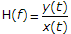then for this to be true x(t) is
exp (j2p f/t)
exp (-j2p f/t)
exp (j2p ft)
exp (-j2p f/t)
Explanation:

If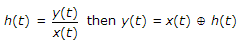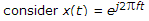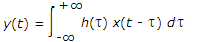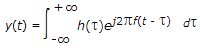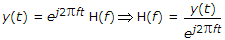.

22.
For the discrete time system of the given figure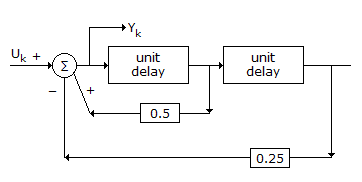yk - 0.5 yk - 1 - 0.25 yk - 2 = uk
yk + 0.5 yk - 1 - 0.25 yk - 2 = uk
yk - 0.5 yk - 1 + 0.25 yk - 2 = uk
yk + 0.5 yk - 1 + 0.25 yk - 2 = uk
Explanation:

uk + 0.5 yk - 1 - 0.25 yk - 2 = yk.

23.
The analog signal m(t) is given below m(t) = 4 cos 100 pt + 8 sin 200 pt + cos 300 pt, the Nyquist sampling rate will be
1/100
1/200
1/300
1/600
Explanation:

m (t) = 4 cos 100 pt + 8 sin 200 pt + cos 300 pt

Nyquist sampling freq fs ≤ 2fm where fm is highest frequency component in given signal and highest fm in 3rd part

2pfmt = 300 pt

fm = 150 Hz

fs = 2 x 150 p 300 Hz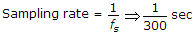.

24.
The ROC of sequence in the Z.T. of sequence x[n] = an ∪ [n] is
z > a
z < a
|z| > a
|z| < a
Explanation:

The simplest method to find the ROC. Put denominator to greater than zero.

25.
In Laplace transform, multiplication by e-at in time domain becomes
translation by a in s domain
translation by (-a) in s domain
multiplication by e-as in s domain
none of the above